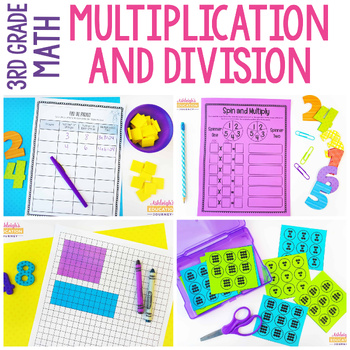# 3rd Grade Multiplication & Division Unit | Print & Digital for Distance Learning3rd
Subjects
Standards
Resource Type
Formats Included
• PDF
•Google Apps™
Pages
150 pagesThe Teacher-Author indicated this resource includes assets from Google Workspace (e.g. docs, slides, etc.).
##### Also included in
1. This yearlong bundle of math workshop resources and lessons includes EVERYTHING you need to implement math workshop in your 3rd grade classroom. These Common Core Math units introduce and practice all third grade math concepts to students through a combination of hands-on activities, skills practice
Price \$65.20Original Price \$81.50Save \$16.30

### Description

This all-inclusive multiplication and division unit has everything you need to teach multiplication and division in 3rd grade. In this product, you will find conceptual lessons, activities, word problems, skill building worksheets, games, and answer keys. You'll also find a detailed pacing guide to help as you plan your multiplication unit.

The skills taught in this unit include representing multiplication through repeated addition, arrays, and grouping models, as well as the properties of multiplication (identity property, associative property, commutative property, and distributive property). There are multiplication word problems, division word problems, and 2-step word problems. The games include I Have Who Has, Multiplication War, and Division Concentration.

Check out these other math units!

Fractions Rock

Linear Measurement

Total Pages
150 pages
Included
Teaching Duration
1 month
Report this resource to TpT
Reported resources will be reviewed by our team. Report this resource to let us know if this resource violates TpT’s content guidelines.

### Standards

to see state-specific standards (only available in the US).
Interpret products of whole numbers, e.g., interpret 5 × 7 as the total number of objects in 5 groups of 7 objects each. For example, describe a context in which a total number of objects can be expressed as 5 × 7.
Interpret whole-number quotients of whole numbers, e.g., interpret 56 ÷ 8 as the number of objects in each share when 56 objects are partitioned equally into 8 shares, or as a number of shares when 56 objects are partitioned into equal shares of 8 objects each. For example, describe a context in which a number of shares or a number of groups can be expressed as 56 ÷ 8.
Use multiplication and division within 100 to solve word problems in situations involving equal groups, arrays, and measurement quantities, e.g., by using drawings and equations with a symbol for the unknown number to represent the problem.
Determine the unknown whole number in a multiplication or division equation relating three whole numbers. For example, determine the unknown number that makes the equation true in each of the equations 8 × ? = 48, 5 = __ ÷ 3, 6 × 6 = ?.
Apply properties of operations as strategies to multiply and divide. Examples: If 6 × 4 = 24 is known, then 4 × 6 = 24 is also known. (Commutative property of multiplication.) 3 × 5 × 2 can be found by 3 × 5 = 15, then 15 × 2 = 30, or by 5 × 2 = 10, then 3 × 10 = 30. (Associative property of multiplication.) Knowing that 8 × 5 = 40 and 8 × 2 = 16, one can find 8 × 7 as 8 × (5 + 2) = (8 × 5) + (8 × 2) = 40 + 16 = 56. (Distributive property.)

### Questions & Answers

Teachers Pay Teachers is an online marketplace where teachers buy and sell original educational materials.